# Right-ordered group

(diff) ← Older revision | Latest revision (diff) | Newer revision → (diff)

A group on whose set of elements a total order(cf. Totally ordered group) is defined such that for allthe inequality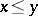implies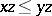. The set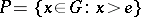of positive elements ofis a pure (i.e.) linear (i.e.) sub-semi-group. Every pure linear sub-semi-groupof an arbitrary group defines a right order, namelyif and only if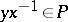.

The group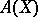of automorphisms of a totally-ordered setcan be right ordered in a natural manner. Every right-ordered group is order-isomorphic to some subgroup offor a suitable totally-ordered set (cf. ). An Archimedean right-ordered group, i.e. a right-ordered group for which Archimedes' axiom holds (cf. Archimedean group), is order-isomorphic to a subgroup of the additive group of real numbers. In contrast with (two-sided) ordered groups, there are non-commutative right-ordered groups without proper convex subgroups (cf. Convex subgroup). The class of right-ordered groups is closed under lexicographic extension. The system of all convex subgroups of a right-ordered groupis totally ordered with respect to inclusion and is complete. This system is solvable (cf. also Solvable group) if and only if for any positive elements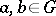there is a natural numbersuch that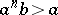. If the group has a solvable subgroup system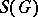whose factors are torsion-free, thencan be right-ordered in such a way that all subgroups inbecome convex. In a locally nilpotent right-ordered group the system of convex subgroups is solvable.

A groupcan be right-ordered if and only if for any finite systemof elements ofthere are numbers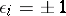,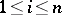, such that the semi-group generated by the set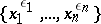does not contain the identity element of.

Every lattice ordering of a groupis the intersection of some of its right-orderings (cf. Lattice-ordered group).

How to Cite This Entry:
Right-ordered group. Encyclopedia of Mathematics. URL: http://encyclopediaofmath.org/index.php?title=Right-ordered_group&oldid=15087
This article was adapted from an original article by V.M. Kopytov (originator), which appeared in Encyclopedia of Mathematics - ISBN 1402006098. See original article